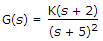# Electronics and Communication Engineering - Automatic Control Systems

Exercise : Automatic Control Systems - Section 10
1.

Assertion (A): If points at infinity are included, G(s) has same number of poles and zeros.

Reason (R): The functionhas a zero at s = - 2.

Both A and R are correct and R is correct explanation of A
Both A and R are correct but R is not correct explanation of A
A is correct but R is wrong
R is correct but A is wrong
Explanation:
No answer description is available. Let's discuss.

2.
If the characteristic equation is (λ + 1) (λ + 2) (λ + 3) = 0, the eigen values are
-1, -2, -3
1, 2, 3
0, 1, 2
0, 2, 3
Explanation:
No answer description is available. Let's discuss.

3.
Lag compensation permits a high gain at low frequencies.
True
False
Explanation:
No answer description is available. Let's discuss.

4.
If the poles lie in second quadrant, damping ratio is
1
less than 1
zero
more than 1
Explanation:
No answer description is available. Let's discuss.

5.

Assertion (A): When performance specifications are given in term of transient response characteristics, root locus method is a suitable method.

Reason (R): When performance specifications in terms of state variables are given, modern control methods can be used.

Both A and R are correct and R is correct explanation of A
Both A and R are correct but R is not correct explanation of A
A is correct but R is wrong
R is correct but A is wrong